Courses

# Test: Permutation And Combination- 3

## 15 Questions MCQ Test General Aptitude for GATE 2020 | Test: Permutation And Combination- 3

Description
This mock test of Test: Permutation And Combination- 3 for GATE helps you for every GATE entrance exam. This contains 15 Multiple Choice Questions for GATE Test: Permutation And Combination- 3 (mcq) to study with solutions a complete question bank. The solved questions answers in this Test: Permutation And Combination- 3 quiz give you a good mix of easy questions and tough questions. GATE students definitely take this Test: Permutation And Combination- 3 exercise for a better result in the exam. You can find other Test: Permutation And Combination- 3 extra questions, long questions & short questions for GATE on EduRev as well by searching above.
QUESTION: 1

### The total number of 9-digit numbers of different digits is

Solution:

It will be a number of 9 digits. 9 x 9 x 8 x 7 x 6 x 5 x 4 x 3 x 2 = 9 x 9!

QUESTION: 2

### Find the number of non-negative integral solutions to the system of equations x + y + z + u + t = 20 and x + y + z = 5.

Solution:

Given, x+y+z+u+t=20...........(1)

and x+y+z=5................(2)

The given system of equations can be written as

u+t=15........(3)

x+y+z=5.............(4)

No. of non-negative integral solutions of (4) are n+r−1​Cr​=3+5−1​C5​=7​C5

No. of non-negative integral solutions of (3) are n+r−1​Cr​=2+15−1​C15​=16​C15

So, required numbers =16​C15​×7C5​=336

QUESTION: 3

### Ateacher takes 3 children from her class to the zoo at a time as often as she can, but she does not take the same three children to the zoo more than once. She finds that she goes to the zoo 84 times more than a particular child goes to the zoo. The number of children in her class is

Solution:

nC3 - n-1C2 = 84, where n is the number of students. Now use options.

QUESTION: 4

If x, y, and z are integers and x ≥ 0, y ≥ 1, z ≥ 2, x+y + z= 15 then the number of values of the ordered triplet (x, y, z) is

Solution:

H ere, Since x ≥ 0 , y ≥ l , z ≥ 2

Now, x can get any number of things, y should get a minimum of 1 thing and z should get a minimum of 2 things. So, let us give these to y and z.
Now , total things left = 12

Now use the formula n identical things can be distributed among r persons in n+x-1Cx-1

Where n = 12, r = 3

QUESTION: 5

In an examination, the question paper contains three different sections A, B and C containing 4, 5 and 6 questions respectively. In how many ways, a candidate can make a selection of 7 questions, selecting at least two questions from each section?

Solution:

Various ways of doing this is as follows: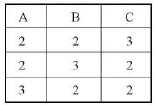Now do the selections.

QUESTION: 6

The number of parallelograms that can be formed from a set of four parallel lines intersecting another set of three parallel lines, is

Solution:

This is nothing but 4C2 x 3C2.

QUESTION: 7

A lady gives a dinner party to 5 guests to be selected from nine friends. The number of ways of forming the party of 5, given that two particular friends A and B will not attend the party together is

Solution:

This can be done in three ways:

i. A is selected but B is not selected - A has already been selected, rest 4 is to be selected from 7 persons = 7C4 = 35

ii. B is selected but A is not selected - 7C4 = 35

iii. Neither A nor B is selected - 7C5 = 21 Total = 91

QUESTION: 8

If a denotes the number of permutations of x + 2 things taking all at a time, b the number of permutations of x things taking 11 at a time and c the number of permutations of x - 11 things taking all at a time such that a = 182be, then the value of x is

Solution: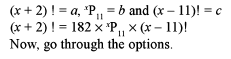QUESTION: 9

How many 10 digits numbers can be written by using the digits 1 and 2

Solution:

2 x 2 x 2 x 2 x 2 x 2 x 2 x 2 x 2 x 2 .

QUESTION: 10

In a room there are 2 green chairs, 3 yellow chairs and 4 blue chairs. In how many ways can Raj choose 3 chairs so that at least one yellow chair is included? ​

Solution: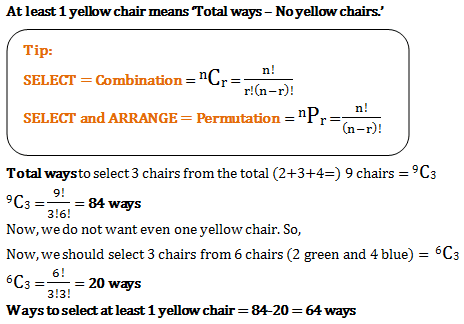QUESTION: 11

Find the number of non-congruent rectangles that can be found on a chessboard normal 8 x 8 chessboard,

Solution:

A chess board is in the shape of a square divided into 8*8 smaller squares of equal dimensions.

If a rectangle formed has one side length 1, then following (length, breadth) combinations possible:-

(1,1), (1, 2), (1, 3), (1, 4), 1,5), (1, 6), (1, 7), (1, 8)

Total 8 non - congruent rectangles.

Similarly with one side as 2 following non - congruent triangles possible:-

(2, 2), (2, 3),.......(2, 8) .
Total 7 non - congurent rectangles.

Similarly for 3,4,5,6,7,8  we get 6,5,4,3,2,1  non - congurent rectangles respectively.

So, total no. Of rectangles will be:-

=> 8+7+6+5+4+3+2+1

=> 36

QUESTION: 12

Find the number of integral solutions of equation

x + y + z + t = 29, x > 0 , y > l , z> 2 and t > 0.

Solution:

This situation is similar to distributing 29 identical things among 4 persons x, y, z and t in such a way that x is getting a minimum 1 thing, y is getting minimum 2 things, z is getting minimum 3 things and t is getting minimum 0 thing.
So, let us distribute 1 thing to x, 2 things to y, 3 things to z and 0 thing to t. After doing this distribution, we are free to distribute the remaining things among x, y, z and t in any ways.
Now the equation is:x + y + z + t = 23 The total number of ways of distributing 23 identical things among 4 persons in such a way that anybody can get any number of things = 26C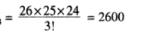QUESTION: 13

There is a regular decagon. Triangles are formed by joining the vertices of the polygon. What is the number of triangles which have no side common with any of the sides of the polygon?

Solution:

. 1. As the first step, let calculate how many triangles can we have connecting all vertices of the decagon. The number of these triangles is exactly equal to the number of combinations of 10 items (vertices) taken 3 at a time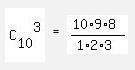= 10*3*4 = 120.

2. Now let exclude from this amount those triangles that have ONLY ONE side of the decagon as their side. The number of excluded triangles is equal to 10*6 = 60 (10 sides of the decagon, and each side may go with one of (10-2-2) = 6 opposite vertices).

3. Last step is to exclude those triangles from 120 of the n.1, that have TWO SIDES of the decagon as their sides. The number of such triangles is 10 (exactly as the number of the decagon's vertices).

4. So, final answer is 120 - 60 - 10 = 50 triangles.

QUESTION: 14

The number of positive integral solution of abc = 30 is:

Solution:

Number of the integral solution for abc=30 are:

1×3×10⇒Permutation=3!

15×2×1⇒Permutation=3!

5×3×2⇒Permutation=3!

5×6×1⇒Permutation=3!

30×1×1⇒Permutation= 3!/2!

Total solutions =(3!×4)+3=27

QUESTION: 15

A dinner menu is to be designed out of 5 different starters, 6 identical main courses and 4 distinct desserts. In how many ways menu be designed such that there is atleast one of each of the starters, main courses and desserts?

Solution: